# Magnetic Equations

Equations

Maxwell equations

Lorentz Force

Magnetic Field

Ampere's Law

Biot-Savart Law

Calculating magnetic forces

# Magnetic Flux Density

Magnetic ﬂux density for a uniformly magnetizied body can be calculated by the formula: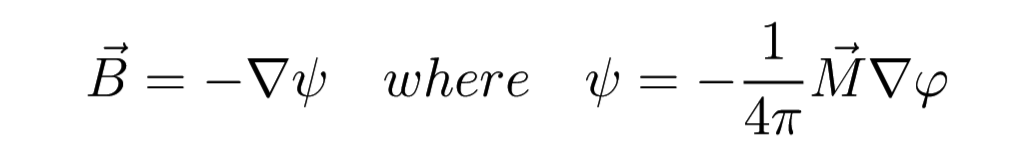(1)

Formula 1 is a scalar potentiel of ﬂux density, M is a constant vector of magnetization, ϕ is a scalar potentiel of the same body charged with unity charge density. The potential can be calculated by the formula:(2)

calculated of the volume of body, Fig:1.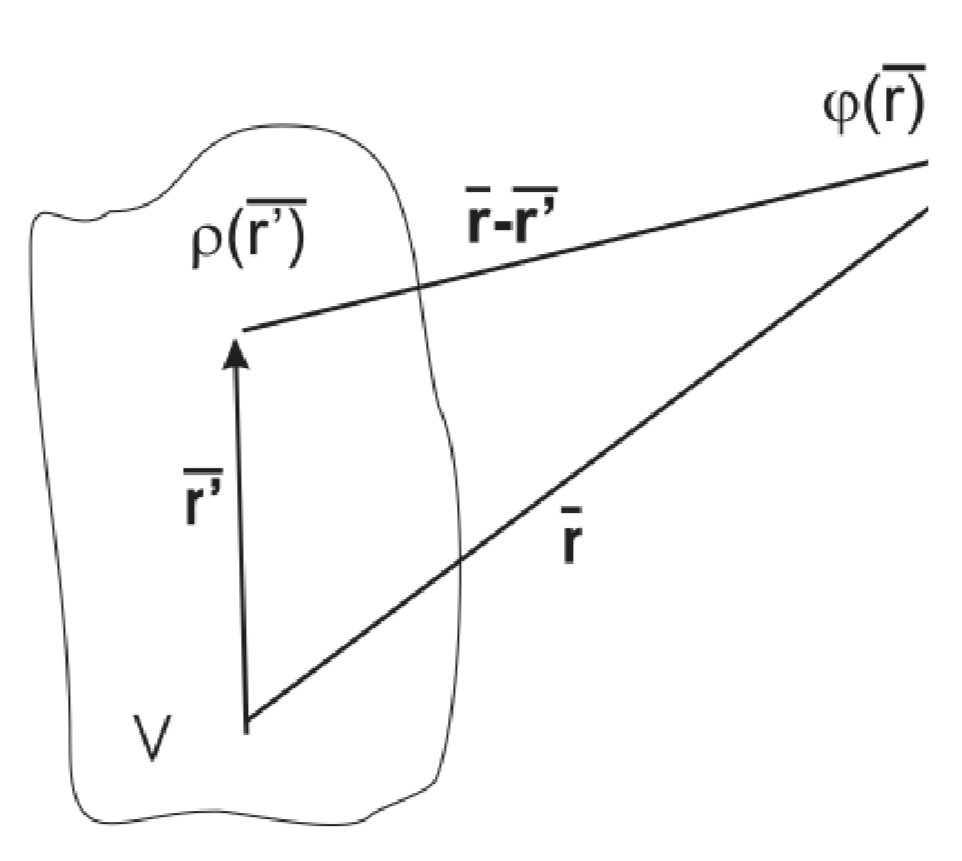Figure 1. Potential Calculation

The volumetric integral calculation is not easy in general so we calculate magnetic ﬂux density only for regular bodies such as blocks/cubes, discs/cylinders, rings and spheres.

Magnetic ﬂux density generated by steady magnets can be calculated by simple formulae only for spherical magnets or for z axis for cylindrical and rectangular magnets.

## Blocks/Cubes

For a block or cube, Fig. 2;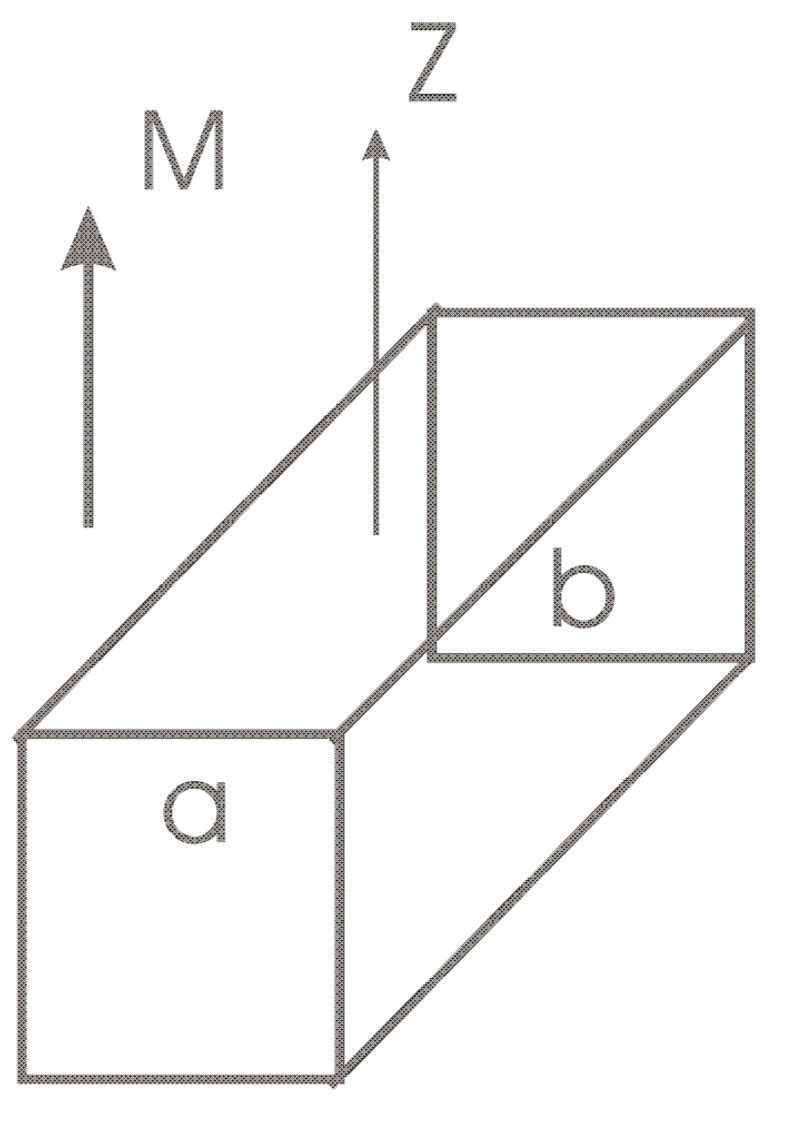Figure 2. Block/Cube

The calculation gives the formulae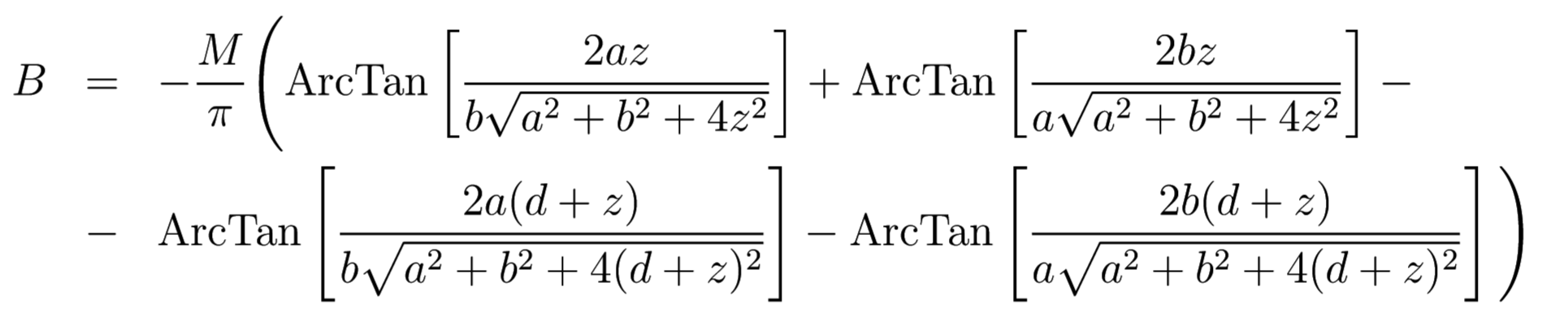(3)

Examples of graphs of magnetic ﬂux density for a rectangle are on Fig.2. The graph is plotted for M=100,a=5mm, b=10mm, d=5mm, range of z is [0.01,10]mm.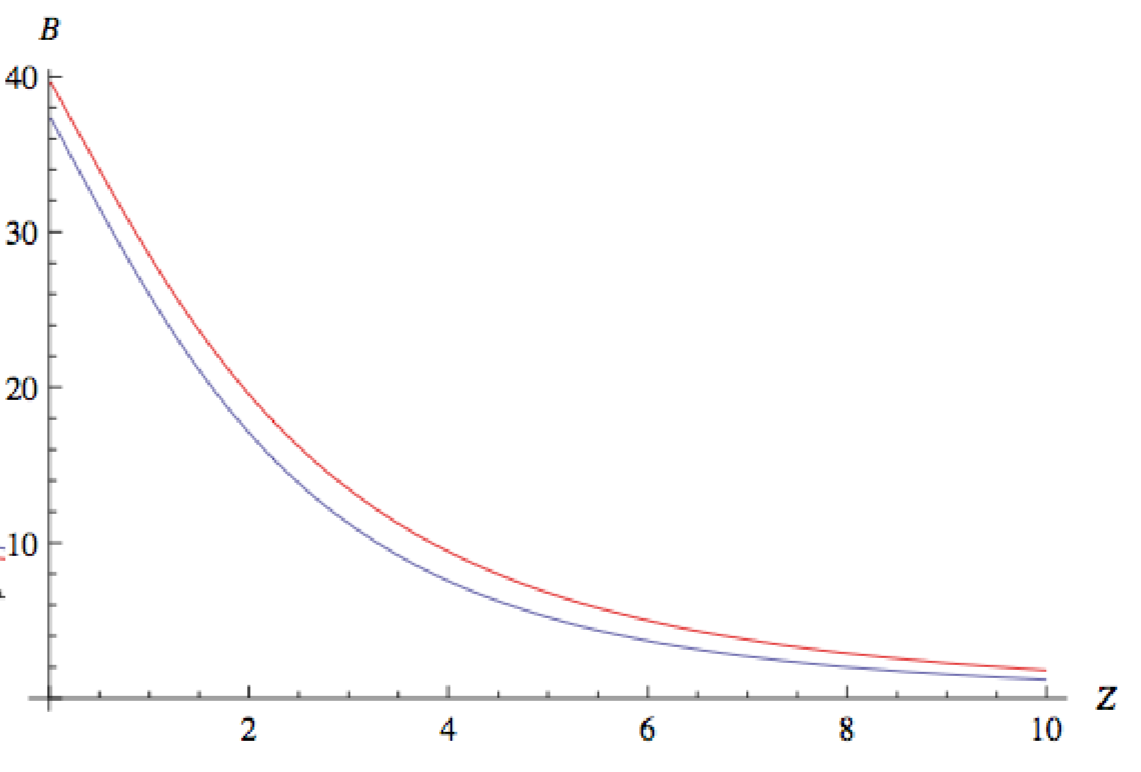Figure 3. Example magnetic flux density with distance

## Disc/Cylinder and Ring

For a disc or cylinder calculation of integrals gives explicit formulae only for z axis, Fig. 4.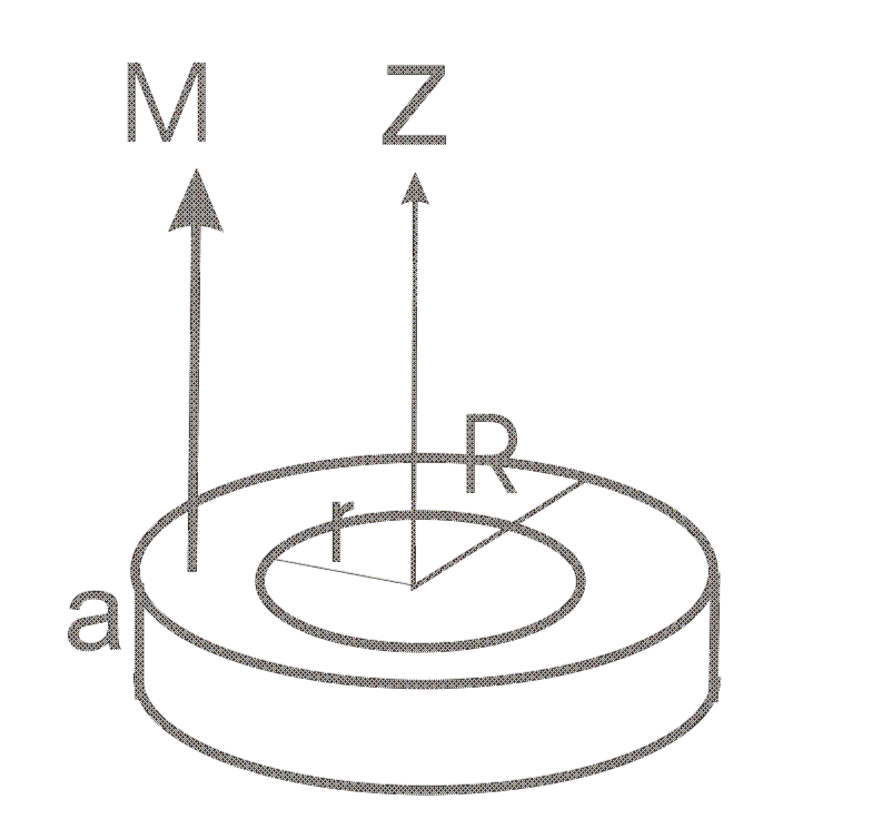Figure 4. Disc/Cylinder and Ring  (for disc/cylinder ignore r)

For disk magnetic ﬂux density on z-axis is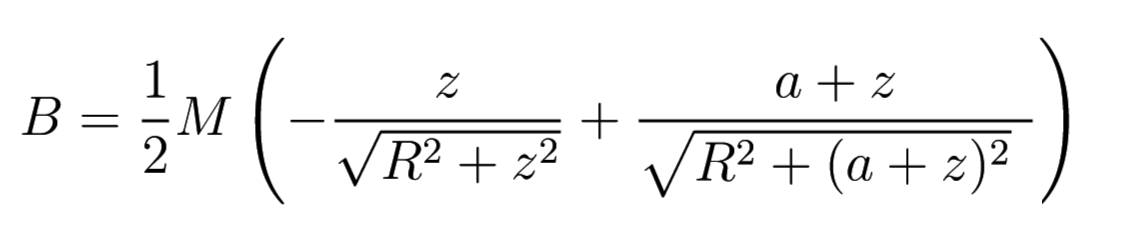(4)

For a ring magnetic ﬂux density on z-axis is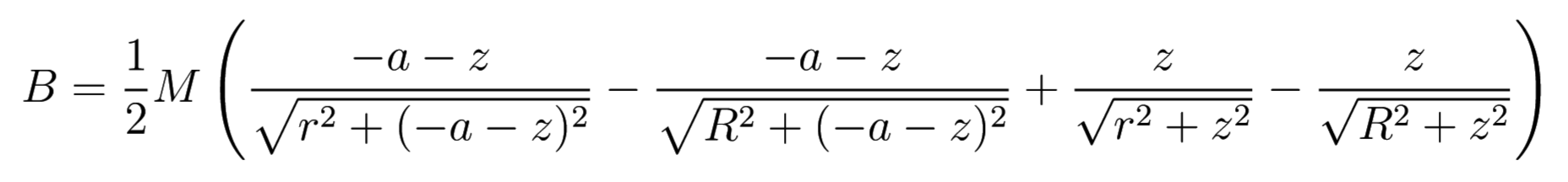(5)

Examples of graphs of magnetic ﬂux density for a ring (red) and a disk (bleu) are on Fig:4. The graph is plotted for M=100,diameter=10mm, height=5mm, range of z is [0.01,10]mm. For the ring an interior diameter=4mm.